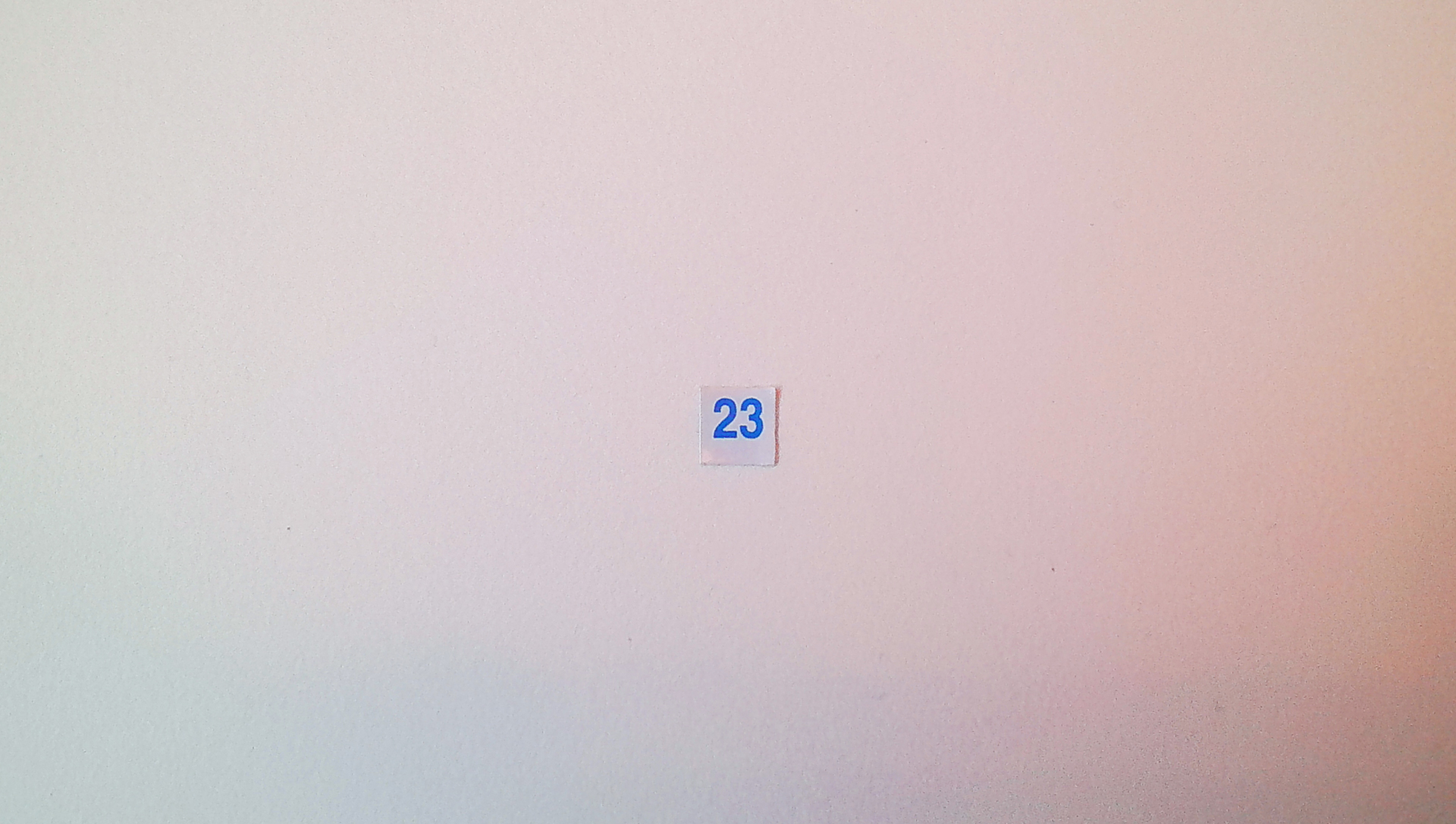### 23

##### 23

23 (twenty-three) is the natural number following 22 and preceding 24.

Twenty-three is the ninth prime number, the smallest odd prime that is not a twin prime. Twenty-three is also the fifth factorial prime, the second Woodall prime. It is an Eisenstein prime with no imaginary part and real part of the form 3n − 1.

23 is the third base-10 repunit prime exponent after 2 and 19.

The fifth Sophie Germain prime and the fourth safe prime, 23 is the next to last member of the first Cunningham chainof the first kind to have five terms (2, 5, 11, 23, 47). Since 14! + 1 is a multiple of 23 but 23 is not one more than a multiple 14, 23 is a Pillai prime. 23 is the smallest odd prime to be a highly cototient number, as the solution to x − φ(x) for the integers 95, 119, 143, 529.

23 is the first prime p for which unique factorization of cyclotomic integers based on the p the root of unity breaks down.

The sum of the first 23 primes is 874, which is divisible by 23, a property shared by few other numbers.

In the list of fortunate numbers, 23 occurs twice, since adding 23 to either the fifth or eighth primorial gives a prime number (namely 2333 and 9699713).

23 also has the distinction of being one of two integers that cannot be expressed as the sum of fewer than 9 cubes of positive integers (the other is 239). See Waring's problem.

23 is a Wedderburn–Etherington number. The codewords in the perfect (non-extended) binary Golay code are of size 23.

According to the birthday paradox, in a group of 23 (or more) randomly chosen people, the probability is more than 50% that some pair of them will have the same birthday. A related coincidence is that 365 times the natural logarithm of 2, approximately 252.999, is very close to the number of pairs of 23 items, 253.

In base 10, 23 is the second Smarandache–Wellin prime, as it is the concatenation of the base 10 representations of the first two primes (2 and 3) and is itself also prime. It is also a happy number in base 10. 23! is 23 digits long in base 10. There are only three other numbers that have this property: 1, 22, and 24.

The natural logarithms of all positive integers lower than 23 are known to have binary BBP-type formulae.

23 is the smallest prime number p such that the largest consecutive pair of p-smooth numbers is the same as the largest consecutive pair of (p − 1)-smooth numbers, as given in the On-Line Encyclopedia of Integer Sequences sequence A228611. That is, the largest consecutive pair of 23-smooth numbers, (11859210, 11859211), is the same as the largest consecutive pair of 22-smooth numbers, and 23 is the smallest prime for which this is true.

23 is the smallest positive solution to Sunzi's original formulation of the Chinese remainder theorem.

##### 2

2 (two) is a number, numeral, and glyph. It is the natural number following 1 and preceding 3.

##### 3

3 (three) is a number, numeral, and glyph. It is the natural number following 2 and preceding 4.

< BACK | NEXT >